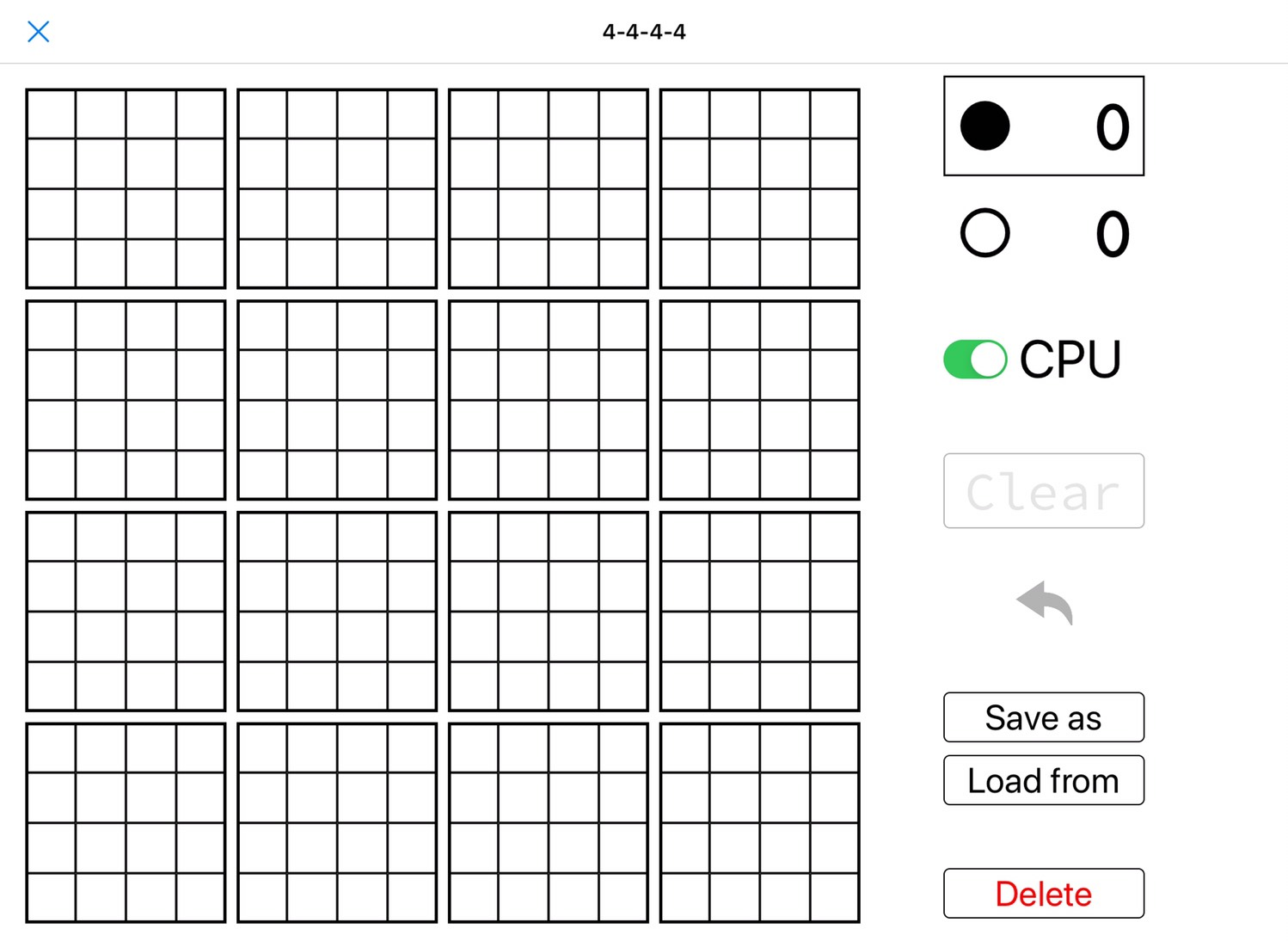# [PYTHON] Let's play with 4D 4th

## Introduction

Do you know the play of three-dimensional four-in-a-row? Try google Somehow you understand As you can see, it is a four-in-a-row arrangement in a 4x4x4 space. I have expanded this to 4 dimensions with ease, so I would like to write about it.

## What was made

I made something like this.I created it on an iPad using Pythonista 3 that runs Python on iOS.

### What you can do

――Play with 4D 4th --Maintaining the board (not reset even when closed) --Saving and loading game records --CPU match

## Implementation story

For the time being, I will mention it on GitHub, but since the environment is limited, I will not discuss the specific UI implementation.

### Aggregate

Since the number of combinations is huge when trying to aggregate seriously, I decided to cover all the sequences by the following procedure.

1. Determine the vector that represents the scanning direction
2. List all suitable coordinates as the scanning start point in that direction.
3. Scan from the starting point in the specified direction

#### 1. Enumeration of direction vectors

For example

--x axis → \$ (1, 0, 0, 0) \$ --Diagonal of y-z plane → \$ (0, \ pm1, 1, 0) \$ --x-z-w Diagonal of cube → \$ (\ pm1, 0, \ pm1, 1) \$ --Diagonal hypercube → \$ (\ pm1, \ pm1, \ pm1, 1) \$

It's like (all multiple issues are optional).

In one-dimensional scanning, the axis of \$ (1, 0, 0, 0) \$ should be shifted, so

``````for i in range(4):
_aggregate([[1, 0, 0, 0][i:]+[1, 0, 0, 0][:i]])
``````

If so, it's OK. (`_Aggregate` is a function that appears after 2)

Scanning in two or more dimensions would make readability sad if a unified implementation was used, so I gave up and wrote all the patterns. I used ʻitertools.product`which returns the direct product to make the compound number optional. For example, for the x-z-w cube, you can represent four diagonals by using`product ([-1, 1], , [-1, 1], )`.

#### 2. Enumeration of starting points

If the scanning direction is +1 for an axis, the coordinates of the starting point must be 0 in order to proceed from 0 → 1 → 2 → 3. On the contrary, if it is -1, it is necessary to start from 3 in order to proceed from 3 → 2 → 1 → 0. If it is 0, then 0, 1, 2, 3 can all be possible. I will write this obediently.

``````def start(val):
if val == 1:
return 
elif val == -1:
return 
else:
return range(4)
``````

#### 3. Scan

Scans in the specified direction from all possible starting points. The starting point is to enumerate all combinations out of multiple for each of the four axes, so ʻitertools.product` comes into play again. Specifically, it looks like this

``````def _aggregate(vec):
for X, Y, Z, W in vec:
if (X, Y, Z, W) == (0, 0, 0, 0):
continue
for x, y, z, w in product(start(X), start(Y), start(Z), start(W)):
s = sum(
self.get_cell(x+X*i, y+Y*i, z+Z*i, w+W*i).player for i in range(4)
)
if s == 4:
self.black += 1
elif s == -4:
self.white += 1
``````

The state of each cell is +1 for ●, -1 for ○, and 0 if nothing is placed, so if you add them all together, you can see that they are complete.

### CPU match

The CPU is currently very simple:

1. If you have 3 stitches, align 4 stitches
2. If you have 3 opponents, stop them
3. For the muscles where the opponent has two eyes, block the parts that are common to many muscles
4. Place the muscles that you have two eyes in the same place as many muscles.
5. If you can't decide on the above four, put it in an open place.

In this game, if you make two 3s at the same time, you win, so there are processes like 3 and 4. (If there are multiple places with the same priority, select them randomly) It is strong for a simple algorithm.

## at the end

If you have Pythonista, please play with it. I would be grateful if anyone could tell me that they had ported it to another environment!